# CBSE Class 10 Science Magnetic Effects Of Current Worksheet Set A

Read and download free pdf of CBSE Class 10 Science Magnetic Effects Of Current Worksheet Set A. Students and teachers of Class 10 Science can get free printable Worksheets for Class 10 Science Chapter 13 Magnetic Effect of Electric Current in PDF format prepared as per the latest syllabus and examination pattern in your schools. Class 10 students should practice questions and answers given here for Science in Class 10 which will help them to improve your knowledge of all important chapters and its topics. Students should also download free pdf of Class 10 Science Worksheets prepared by school teachers as per the latest NCERT, CBSE, KVS books and syllabus issued this academic year and solve important problems with solutions on daily basis to get more score in school exams and tests

## Worksheet for Class 10 Science Chapter 13 Magnetic Effect of Electric Current

Class 10 Science students should refer to the following printable worksheet in Pdf for Chapter 13 Magnetic Effect of Electric Current in Class 10. This test paper with questions and answers for Class 10 will be very useful for exams and help you to score good marks

### Class 10 Science Worksheet for Chapter 13 Magnetic Effect of Electric Current

MCQ Questions for NCERT Class 10 Science Magnetic Effects Of Electric Current

Question. Calculate the magnetic field produced by the solenoid of length 50 cm with no. of turns in the coil 210 when the current passing through it 8 A. (Given permeability, μ0 = 4p × 10– 7 Wb/Am)
(a) 4.22 × 10– 7 T
(b) 42.24 × 10– 7 T
(c) 422.2 × 10–7 T
(d) 422.2 × 10– 5 T

Question. The figure given below shows the magnetic field produced by a currents carrying wire. Which of the diagram shows it correctly?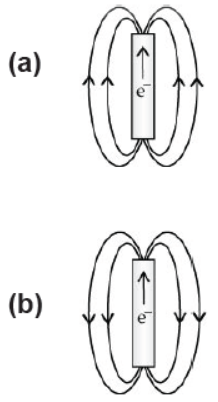Question. Commercial motors do not use:
(a) an electromagnet to rotate the armature
(b) effectively large number of turns of conducting wire in the current carrying coil
(c) a permanent magnet to rotate the armature
(d) a soft iron core on which the coil is wound

Question. Fleming's Right-hand rule gives:
(a) Magnitude of the induced current.
(b) Magnitude of the magnetic field.
(c) Direction of the induced current.
(d) Both, direction and magnitude of the induced current.

Question. The magnetic field inside the solenoid is:
(a) non-uniform
(b) variable
(c) same at all points
(d) zero

Question. A bar magnet is immersed in a heap of iron fillings and pulled out. The amount of iron filling dinging to the:
(a) North pole is almost equal to the South pole
(b) North pole is much more than the South Pole
(c) North pole is almost less equal than the South pole
(d) Magnet will be same all along its length

Question. Which of the following property of a proton can change while it moves freely in a magnetic field?
(a) Mass
(b) Speed
(c) Velocity
(d) Charge

Question. Pick out the incorrect statement about magnetic lines of force.
(a) Magnetic lines of forces start from the North Pole and end on the South Pole.
(b) No two magnetic lines of force can intersect each other.
(b) Magnetic lines of force are far away from each other at the poles.
(d) Magnetic lines of force are closed continuous curves.

Question. Which one of the following substances is the magnetic substances?
(a) Mercury
(b) Iron
(c) Gold
(d) Silver

Question. The figure below shows the Fleming's left hand rule. Identify the correct label with the function?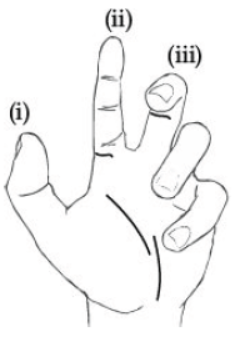(a) Thumb force
(b) Fore finger field
(c) Middle finger-correct
(d) All of these

Question : The phenomena of electromagnetic induction is
(a) the process of generating magnetic field due to a current passing through a coil
(b) the process of charging a body
(c) the process of rotating coil of an electric motor.
(d) producing induced current in a coil by the relative motion between a magnet and the coil

Explanation: When a straight coil and a magnet are moved relative to each other, a current is induced in the coil. This phenomenon is known as electromagnetic induction.

Question : Which one of the following correctly describes the magnetic field near a long straight wire?
(a) The field consists of straight lines parallel to the wire
(b) The field consists of concentric circles centered on the wire
(c) The field consists of straight lines perpendicular to the wire
(d) The field consists of radial lines originating from the wire

Explanation: The field consists of concentric circles centered on the wire.It originates from the cicumference of the wire and spread out thus creating concentric circles

Question : The device used for producing electric current is called
(a) galvanometer
(b) generator
(c) ammeter
(d) motor

Explanation: Electric current is produced by electric generator which converts mechanical energy into electricity.

Question : Match the following with correct response.
(2) Magnetic flux                                                (B) Electrical energy into mechanical energy
(3) Electric motor                                               (C) Number of magnetic lines passing normally through the surface
(4) Permanent magnet                                        (D) Bar magnet
(a) 1-A, 2-C, 3-B, 4-D
(b) 1-B, 2-D, 3-A, 4-C
(c) 1-C, 2-B, 3-D, 4-A
(d) 1-D, 2-A, 3-C, 4-B

Explanation:
i. A lodestone is a naturally magnetized piece of the mineral magnetite. Loadstone is iron ore.
ii. Magnetic flux is the number of magnetic field lines passing through a surface placed in a magnetic field.
iii. An electric motor converts electrical energy into mechanical energy.
iv. Permanent magnet are usually made of hard materials which are strongly magnetized. A perfect example of permanent magnet is the Bar Magnet. In fact, Permanent magnets are most of the times referred to as Bar Magnets also.

Question : Which rule determines the direction of flow of current in the conductor?
(a) Fleming’s left hand rule
(b) Fleming’s right hand rule
(c) Maxwell's right hand grip rule
(d) Left hand thumb rule

Explanation: The direction of induced current in a straight conductor is given by Fleming’s right hand rule.
It states that if we stretch the thumb, forefinger and the middle finger of right hand at right angles to one another in such a way that the forefinger points in the direction of magnetic field.
Then, thumb gives the direction of motion of conductor (force), forefinger indicates direction of magnetic field and the middle finger points the direction of induced current.

Assertion and Reasoning Based Questions :

Question. Assertion: A current carrying conductor experiences a force in a magnetic field.
Reason: The net charge on a current carrying conductor is zero.
(a) If both assertion and reason are true and reason is the correct explanation of assertion.
(b) If both assertion and reason are true, but reason is not the correct explanation of assertion.
(c) If assertion is true, but reason is false.
(d) If assertion is false, but reason is true.

Question. Assertion: Magnetic field lines do not intersect each other.
Reason: There are two direction of the magnetic field at a point.
(a) If both assertion and reason are true and reason is the correct explanation of assertion.
(b) If both assertion and reason are true, but reason is not the correct explanation of assertion.
(c) If assertion is true, but reason is false.
(d) If assertion is false, but reason is true.

Question. Assertion: The energy of charged particle moving in a uniform magnetic field does not change.
Reason: Work done by magnetic field on the charge is zero.
(a) If both assertion and reason are true and reason is the correct explanation of assertion.
(b) If both assertion and reason are true, but reason is not the correct explanation of assertion.
(c) If assertion is true, but reason is false.
(d) If assertion is false, but reason is true.

Question. Assertion: The principle of electromagnetic induction was discovered by Micheal Faraday
Reason: The principle is used only in DC generators
(a) If both assertion and reason are true and reason is the correct explanation of assertion.
(b) If both assertion and reason are true, but reason is not the correct explanation of assertion.
(c) If assertion is true, but reason is false.
(d) If assertion is false, but reason is true.

Question. Assertion: A pump operated by electric motor starts pumping liquid.
Reason: Motor converts mechanical energy to electrical energy.
(a) If both assertion and reason are true and reason is the correct explanation of assertion.
(b) If both assertion and reason are true, but reason is not the correct explanation of assertion.
(c) If assertion is true, but reason is false.
(d) If assertion is false, but reason is true.

Question. Why are magnetic field lines closed curves?
Answer :  The magnetic field lines are closed curves because magnetic field lines originate from the north pole of a magnet and end at its south pole and inside the magnet, it is directed from south pole to north pole.

Question. Name the type of current: (a) used in household supply (b) given by a cell.
Answer :  a. We use alternating current in our houses which changes to direction and magnitude with time.
b. A cell supply direct current (DC) or unidirectional current which flows only in one direction only.

Question. Suggest one way of discriminating a wire carrying current from a wire carrying no current.
Answer :  When a magnetic compass is brought near a current carrying conductor it will be deflected. If magnetic compass remains undeflected near a conductor then there is no current in it.

Question. Write any one method to induce current in a coil.
Answer :  By moving a magnet towards the coil or vice versa, current can be induced in the coil.

Question. State the rule which you use to find the direction of induced current or state Fleming’s right hand rule.
Answer :  Fleming’s right hand rule gives the direction of current produced in a conductor. According to Fleming’s right hand rule, if we stretch right hand thumb, forefinger and middle finger perpendicular to each other in such a way that thumb points the direction of force on a conductor and forefinger points the direction of magnetic field then middle finger will point the direction of current produced in the conductor.

Question. State the direction of magnetic field in the following case:Answer :  Perpendicular to both current and force on the conductor outward the plane of paper.

Question. Why are magnetic field lines closed curves?
Answer :  By convention the magnetic field lines are the path traced by north pole which emerges from north pole and goes to the south pole and inside the magnet the direction of field lines are from south pole to north pole, forming continuous closed path.

Question. How can it be shown that a magnetic field exists around a wire through which a direct current is passing.
Answer :  When we put a small magnetic compass near a current carrying conductor it gets deflected, which shows that a magnetic field is produced near a current carrying conductor.

Question. What type of core is used to make an electromagnet?
Ans : Soft iron core is used in making an electromagnet.

Question. What will be the frequency of an alternating current if its direction changes after every 0.01 s?
Answer :  The direction of ac changes every 0.01 sec.
Thus time of one complete cycle = 0.02 sec
i.e. in 0.02 sec no. of cycle = 1
in 1 sec no. of cycle = 1/0.02 = 100/2 = 50
Frequency of ac = 50 Hz

Question. Mention the special feature regarding shape of magnetic field lines.
Answer :  Magnetic field lines are continuous closed loops.

Question. What constitutes the field of a magnet?
Answer :  The magnetic field is created by the magnet or when a charge particle is moving with some velocity in both cases they produce a magnetic field around them. The magnetic field is due to current or magnetic material.

Question. How is the strength of the magnetic field at a point near a wire related to the strength of the electric current flowing in the wire?
Answer :  The magnetic field strength at a point near a wire is directly proportional to the current strength in the conductor.

Question. State the direction of the magnetic field inside the bar magnet.
Answer :  In a magnet, magnetic field lines goes from S pole to N pole.

Question. Mention the voltage and frequency of current that we receive at our house.
Answer :  In our house we get AC of voltage 220 V and frequency 50 Hz.

Question. What does the thumb indicated in Fleming’s right hand rule?
Answer :  Thumb indicate the direction of force on conductor i.e. motion of the conductor.

Question. If field lines of a magnetic field are crossed at a point, what does it indicate?
Answer :  If the magnetic field lines would cross each other then at the same point there would be two directions of magnetic field which is not possible.

Question. Draw a diagram to represent a uniform magnetic field in a given region.
Answer :  Uniform magnetic field is represented by equidistant parallel lines.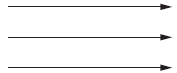Question. When is the force experienced by a current carrying conductor placed in a magnetic field the maximum?
Answer :  A current carrying conductor experience maximum force in a magnetic field when the direction of current is perpendicular to the magnetic field.

Question. An alternating electric current has a frequency of 50 Hz. How many times does it change its direction in one second?
Answer :  50 Hz ac changes its direction 100 times in 1 sec.

Question. How can you show that the magnetic field produced by a given electric current in the wire decreases as the distance from the wire decreases?
Answer :  If we bring a magnetic compass from a distance to near a current carrying conductor its deflection goes on increasing and when magnetic compass is brought away from the current carrying wire its deflection goes on decreasing which shows that magnetic field near current carrying wire is maximum and decreasing on increasing the separation.

Question. Mention the shape of the magnetic field lines around a current carrying straight conductor.
Answer :  The magnetic field lines around a current carrying straight conductor are in the form of concentric circular rings around a conductor.Question. What is the pattern of field fines inside a solenoid? What do they indicate?
Answer :  The magnetic field inside a solenoid is uniform in the form of parallel lines.

Question. How is the magnetic field produced in a solenoid used?
Answer :  Magnetic field produced in a solenoid can be used in making a soft iron piece electromagnet.

Question. What is short-circuiting in an electric supply?
Answer :  When a neutral wire and live wire come in contact with each other short circuit takes place.

Question. What does the direction of thumb indicate in the right-hand thumb rule?
Answer :  Thumb points the direction of current in the conductor holding a straight conductor in right hand.

Question. State the observation made by Oersted on the basis of his experiment with current carrying conductors.
Answer :  A magnetic field is produced near a current carrying conductor which last so long till there is current in the conductor on reversing the current the direction of magnetic field is also reversed.

Question. How is the induced current in a secondary coil related to current in a primary coil?
Ans : Induced current in a secondary coil may be more or lesser than the current in primary coil depending upon the number of turns in secondary.

Question. What is the advantage of the third wire of earth connection in domestic appliances?
Answer :  In case of any electric fault in domestic appliances, current may comes in appliance body. The third wire called earth wire transfer this current to the earth and user remains safe from any such electric shock.

Question. Mention the angle between a current carrying conductor and magnetic field for which the force experienced by this current carrying conductor placed in magnetic field is largest?
Answer :  If the angle between a current carrying conductor and magnetic field is 90° then the force experienced by the conductor is maximum.

Question.  What is the principle of an electric motor ?
Answer :   Electric motor is based upon Fleming's left hand rule.

Answer :  The supply wires as well the wires used in household wiring has a specific rating. The rating of 15A means that if a current upto 15A is passed through circuit, there is no likely damaged feared to the circuit. But if a current more than maximum allowed limit is passed, there may be excessive heating of the wires and it may damage the wiring due to excessive heating.

Question. How should the electric lamps in a building be connected, so that the switching on or off in a room has no effect on other lamps in the same building?
Answer :  All the lamps should be connected in parallel.

Question. Draw magnetic lines around a bar magnet.
Answer :  Take a small compass and a bar magnet. Place it on a sheet of paper fixed on drawing board by using adhesive tape. Mark the boundary of the magnet. Place compass near north pole of the magnet. South pole of compass will point towards north pole of the magnet. Mark the two points a, b of the needle. Move the needle to a new position such that S of compass is at b [position previously held by N]. Proceed till you reach south pole of the magnet. Joint the marked points on a paper by a smooth line which gives one magnetic line of force. Repeat the procedure taking different starting points.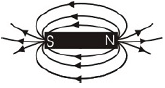Magnetic field around a bar magnet
It will be found that no two magnetic lines of force intersect each other. Relative strength of magnetic field is shown by the degree of closers of the field lines. It is more when field is crowded and weak when field lines are at distance.

Question : Mahesh bought an electric iron and connected its wires into the two-pin plug.
Obviously, the green wire was not connected anywhere. Few days later, his wife got a severe electric shock while ironing the clothes. The electrician told Mahesh that this situation could be averted, if he had connected the green wire also, using the threepin plug. Mahesh learnt a lesson for a life-time.
i. Which terminal was to be connected using green wire?
ii. What qualities does Mahesh need to incorporate in himself to avoid such
mistakes?
iii. If you were the electrician, what else would you do than explaining to Mahesh?
Answer :  i. The terminal to be connected by green wire is the earthing terminal.
ii. Mahesh needs to be more careful, concerned about safety measures and be less ignorant about simple safety practices.
iii. I would have checked all other devices to ensure the further safety.

Question : Explain the meanings of words "electromagnetic" and "induction" in the term electromagnetic induction. List three factors on which the value of induced current produced in a circuit depends.
Name and state the rule used to determine the direction of induced current. State one practical application of this phenomenon in everyday life.
Answer : The word "electromagnetic" is related to the interrelation between electric current I and magnetic field B. While "Induction" is the process of giving rise to something. So the process of generation of an electric current I from magnetic effects B is called electro - magnetic induction.
Three factors which affects the electro-magnetic induction are:
Fleming's right-hand rule used to determine the direction of induced current.
Electric generator is based on the principle of electromagnetic induction.
i. The number of turns in a coil
ii. The strength of magnet used and
iii. The speed by which magnet is pushed into the coil.

Question : Consider a circular loop of wire lying in the plane of the table. Let the current pass through the loop in the clockwise direction. Apply right-hand rule to find out the direction of the magnetic field inside and outside the loop.
Answer : Since the current passes through the loop in clockwise direction, therefore, the front face of the loop will be the south pole and the back face, i.e., the face touching the table will be north pole. According to right-hand rule, the direction of magnetic field inside the loop will be pointing downward. Outside the loop, the direction of the magnetic field will be upward.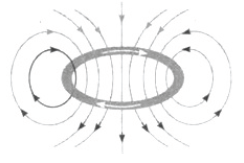Question : For the current carrying solenoid, draw magnetic field lines and give reason to explain that out of the three points A, B and C at which point the field strength is maximum and at which point it is minimum.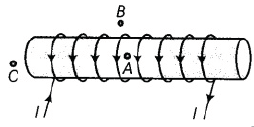Answer :   Magnetic field lines due to a solenoid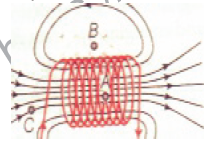In case of an ideal solenoid, magnetic field strength is maximum at point A and is minimum or zero at point B. This is because the magnetic field is strong, where magnetic field lines are far apart. At the point, C, the density of the field lines is less than that of point A , but greater than that of point B. So, the order of the magnetic field at points A, B and C is  BB < BC < BA

Question : Draw a labelled diagram of an electric motor. Explain its principle and working. What is the function of split ring in an electric motor?
Answer :   Electric motor labelled diagram of an electric motor is as follows:Principle: A current-carrying conductor, when placed in a magnetic field, experiences a force. If the direction of magnetic field and that of current are mutually perpendicular, then force acting on the conductor will be perpendicular to both and will be the given by Fleming’s left-hand rule. Due to this force the conductor begins to move, if it is free to rotate.
Working: Let the current in the coil ABCD of motor enters from the source battery through the conducting brush X, flow along ABCD and finally flows back to the battery through brush Y. On applying Fleming’s left-hand rule we find that force acting on arm AB due to magnetic field pushes it downwards. But the force acting on arm CD pushes it upwards. Thus, the coil and the axle rotate anticlockwise. Due to action of split rings P and Q change their contacts with brushes. Now, P makes contact with Y and Q with X. As a result, Current begins to flow in coil along DCBA. The arms are pushed in opposite direction and coil continues to rotate in same direction.

Question. A coil of insulated copper wire is connected to a galvanometer. What will happen if a bar magnet is (i) pushed into the coil, (ii) withdrawn from inside the coil, (iii) held stationary inside the coil?
Answer : (i) When a bar magnet is pushed into the coil, current is induced in the coil momentarily as a result the galvanometer deflects in a particular direction momentarily.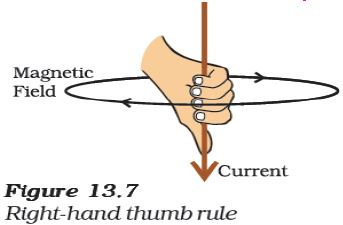(ii) When the bar magnet is withdrawn from inside the coil, current is induced momentarily but in the opposite direction and the galvanometer deflects in the opposite direction momentarily.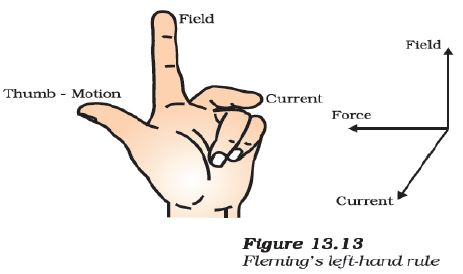(iii) When the bar magnet is held stationary inside the coil, no current will be induced as a result there will be no deflection in the galvanometer.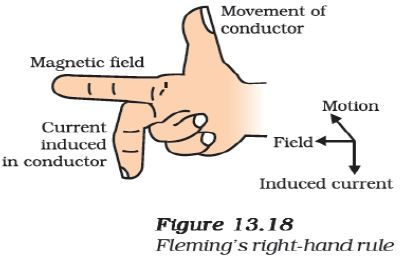## More Study Material

### CBSE Class 10 Science Chapter 13 Magnetic Effect of Electric Current Worksheet

We hope students liked the above worksheet for Chapter 13 Magnetic Effect of Electric Current designed as per the latest syllabus for Class 10 Science released by CBSE. Students of Class 10 should download in Pdf format and practice the questions and solutions given in the above worksheet for Class 10 Science on a daily basis. All the latest worksheets with answers have been developed for Science by referring to the most important and regularly asked topics that the students should learn and practice to get better scores in their class tests and examinations. Studiestoday is the best portal for Class 10 students to get all the latest study material free of cost.

### Worksheet for Science CBSE Class 10 Chapter 13 Magnetic Effect of Electric Current

Expert teachers of studiestoday have referred to the NCERT book for Class 10 Science to develop the Science Class 10 worksheet. If you download the practice worksheet for one chapter daily, you will get higher and better marks in Class 10 exams this year as you will have stronger concepts. Daily questions practice of Science worksheet and its study material will help students to have a stronger understanding of all concepts and also make them experts on all scoring topics. You can easily download and save all revision worksheet for Class 10 Science also from www.studiestoday.com without paying anything in Pdf format. After solving the questions given in the worksheet which have been developed as per the latest course books also refer to the NCERT solutions for Class 10 Science designed by our teachers

#### Chapter 13 Magnetic Effect of Electric Current worksheet Science CBSE Class 10

All worksheets given above for Class 10 Science have been made as per the latest syllabus and books issued for the current academic year. The students of Class 10 can be rest assured that the answers have been also provided by our teachers for all worksheet of Science so that you are able to solve the questions and then compare your answers with the solutions provided by us. We have also provided a lot of MCQ questions for Class 10 Science in the worksheet so that you can solve questions relating to all topics given in each chapter. All study material for Class 10 Science students have been given on studiestoday.

#### Chapter 13 Magnetic Effect of Electric Current CBSE Class 10 Science Worksheet

Regular worksheet practice helps to gain more practice in solving questions to obtain a more comprehensive understanding of Chapter 13 Magnetic Effect of Electric Current concepts. Worksheets play an important role in developing an understanding of Chapter 13 Magnetic Effect of Electric Current in CBSE Class 10. Students can download and save or print all the worksheets, printable assignments, and practice sheets of the above chapter in Class 10 Science in Pdf format from studiestoday. You can print or read them online on your computer or mobile or any other device. After solving these you should also refer to Class 10 Science MCQ Test for the same chapter.

#### Worksheet for CBSE Science Class 10 Chapter 13 Magnetic Effect of Electric Current

CBSE Class 10 Science best textbooks have been used for writing the problems given in the above worksheet. If you have tests coming up then you should revise all concepts relating to Chapter 13 Magnetic Effect of Electric Current and then take out a print of the above worksheet and attempt all problems. We have also provided a lot of other Worksheets for Class 10 Science which you can use to further make yourself better in Science

Where can I download latest CBSE Printable worksheets for Class 10 Science Chapter 13 Magnetic Effect of Electric Current

You can download the CBSE Printable worksheets for Class 10 Science Chapter 13 Magnetic Effect of Electric Current for latest session from StudiesToday.com

Can I download the Printable worksheets of Chapter 13 Magnetic Effect of Electric Current Class 10 Science in Pdf

Yes, you can click on the links above and download Printable worksheets in PDFs for Chapter 13 Magnetic Effect of Electric Current Class 10 for Science

Are the Class 10 Science Chapter 13 Magnetic Effect of Electric Current Printable worksheets available for the latest session

Yes, the Printable worksheets issued for Class 10 Science Chapter 13 Magnetic Effect of Electric Current have been made available here for latest academic session

How can I download the Class 10 Science Chapter 13 Magnetic Effect of Electric Current Printable worksheets

You can easily access the links above and download the Class 10 Printable worksheets Science Chapter 13 Magnetic Effect of Electric Current for each chapter

Is there any charge for the Printable worksheets for Class 10 Science Chapter 13 Magnetic Effect of Electric Current

There is no charge for the Printable worksheets for Class 10 CBSE Science Chapter 13 Magnetic Effect of Electric Current you can download everything free

How can I improve my scores by solving questions given in Printable worksheets in Class 10 Science Chapter 13 Magnetic Effect of Electric Current

Regular revision of practice worksheets given on studiestoday for Class 10 subject Science Chapter 13 Magnetic Effect of Electric Current can help you to score better marks in exams

Are there any websites that offer free test sheets for Class 10 Science Chapter 13 Magnetic Effect of Electric Current

Yes, studiestoday.com provides all latest NCERT Chapter 13 Magnetic Effect of Electric Current Class 10 Science test sheets with answers based on the latest books for the current academic session

Can test papers for Class 10 Science Chapter 13 Magnetic Effect of Electric Current be accessed on mobile devices

Yes, studiestoday provides worksheets in Pdf for Chapter 13 Magnetic Effect of Electric Current Class 10 Science in mobile-friendly format and can be accessed on smartphones and tablets.

Are worksheets for Chapter 13 Magnetic Effect of Electric Current Class 10 Science available in multiple languages

Yes, worksheets for Chapter 13 Magnetic Effect of Electric Current Class 10 Science are available in multiple languages, including English, Hindi